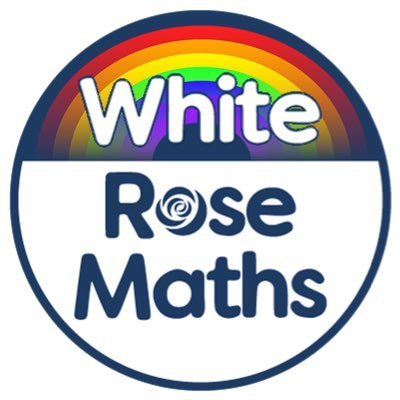Here are this week’s mathematics resources (1st – 5th June) from White Rose Maths:

Y3 worksheets

Y4 worksheets

Y5 worksheets

Y6 worksheetsHere are the mathematics resources from before half term (18th – 22nd May) from White Rose Maths:

Y3 worksheets

Y4 worksheets